Localization and inflation have become essential means of mitigating the effects of the low-rank approximation in ensemble methods. Localization increases the effective rank of the ensemble covariance matrix and allows it to fit a large number of independent observations. Thus, we use localization to reduce sampling errors, in combination with inflation, to reduce the underestimation of the ensemble variance caused by the low-rank approximation. These methods are essential for high-dimensional applications, and this chapter will give a general introduction to various formulations of localization and inflation methods.

## 1 Background

The accuracy of data-assimilation methods that exploit a Gaussian prior is strongly dependent on the quality of the prior covariance matrix. This fact is actual both for variational and for ensemble Kalman filters. The prior EnKF ensemble is genuinely a low-rank approximation, and localization is a way to increase the rank of that matrix drastically. That is essential for being able to fit a large number of independent observations. Furthermore, we also use localization to reduce sampling errors, in combination with a technique called inflation, which is essential for high-dimensional applications. The ensemble approximation is not severe when using a sufficiently large ensemble, and we would happily accept the updated ensemble as our posterior estimate. However, we are often limited to using few realizations for computational reasons, limiting the available range of the solution space and leading to significant sampling errors. For example, the Topaz ocean prediction system described by  Xie et al. (2017) currently has $$\mathcal {O}(10^8)$$ state variables and assimilates $$\mathcal {O}(10^5)$$ measurements in each update step while the ensemble size is 100. Thus, although the “effective” dimension of the model state vector is less than $$\mathcal {O}(10^8)$$, the state space is vastly undersampled, and the ensemble size is too small to represent all the information provided by a large number of measurements. We would not be able to run these high-dimensional systems without localization and inflation.

Suppose the measurements contain spatial variability on scales that the model cannot simulate. In that case, localization allows introducing these finer scales in the updated ensemble and obtaining a model that better fits the observations. It then becomes a partly philosophical and partly computational question whether it makes sense to introduce these fine scales in the model or not. The alternative would be to treat the finer scales in the measurements as representation errors. We can then reduce the measurements’ impact by increasing their error variance and specifying correlated measurement errors. Still, we need localization in large-scale systems since the ensemble space cannot generally accommodate all the information provided by the measurements.

We also use localization in particle filters, but for a different reason. There the issue is filter degeneracy and weight collapse due to a too large number of independent observations. Localization limits the number of measurements each gridpoint sees, reducing the degeneracy problem. This chapter provides the basis for different localization methods and formulations, including Kalman gain localization, covariance localization, and local analysis. We will see that localization and inflation are well developed for the EnKF, while it remains a severe obstacle in particle filtering.

## 2 Various Forms of the EnKF Update

This section contains various forms of the EnKF update equations that we will use throughout this chapter. With reference to definitions given in Chap. 8, we can write the EnKF update Eq. (8.29), in the following alternative but equivalent forms: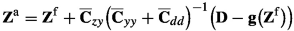(10.1)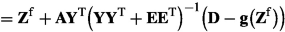(10.2)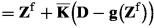(10.3)
\begin{aligned} &= {\mathbf {Z}}^{\mathrm {f}}+ \overline{{\mathbf {C}}}_{zy} {\mathbf {B}} \end{aligned}
(10.4)
\begin{aligned} &= {\mathbf {Z}}^{\mathrm {f}}+ {\mathbf {A}}{\mathbf {W}} \end{aligned}
(10.5)
\begin{aligned} &= {\mathbf {Z}}^{\mathrm {f}}\overline{{\mathbf {T}}}. \end{aligned}
(10.6)

Here each column of $${\mathbf {Z}}$$ represents an ensemble realization, and the corresponding column in $${\mathbf {D}}- {\mathbf {g}}\bigl ({\mathbf {Z}}^{\mathrm {f}}\bigr )$$ is this realization’s innovation vector, i.e., the difference between perturbed and predicted observations. The various representations of the EnKF update allow for making different interpretations of it’s ensemble low-rank approximations. We eliminate apparence of the projection $${\mathbf {A}}^\dagger {\mathbf {A}}$$ from the analysis equations by using Eq. (8.7) in the following discussion.

In Eqs. (10.2) and (10.5) we have used Eq. (8.32).

In Eq. (10.3) we have defined the ensemble representation of the Kalman gain from Eq. (6.39) as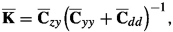(10.7)

which leads to an interpretation of the analysis as computing the update as linear combinations of the m columns of the Kalman gain matrix.

The representer update in Eq. (10.4) is the ensemble version of the formulation in Eqs. (6.40)–(6.42). We can interpret the representer update in Eq. (10.4) as computing the update by adding a linear combination of covariance functions (or representer functions), one for each measurement, to the prior. Thus, $$\overline{{\mathbf {C}}}_{zy}$$ is the representer functions’ ensemble representation and the columns in $${\mathbf {B}}$$ define the linear combinations of representer functions used to create the analyzed ensemble members. The matrix $${\mathbf {B}}$$ is the solution of the following m-dimensional linear system of equations with N right-hand sides,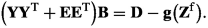(10.8)

Thus, both the representer formulation and the Kalman gain version of the analysis update have a similar interpretation. Both Eqs. (10.3) and (10.4) computes the solution in observation space as defined by the dimension of the matrix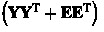. However, in the case with $$N< m$$ the update is still of low rank and we can compute it more efficiently using Eqs. (10.5) or (10.6).

## 3 Impact of Sampling Errors in the EnKF Update

There are three major consequences of using a low-rank ensemble approximation when computing the analysis updates and we will discuss each of them in this section.

### 3.1 Spurious Correlations

Initially, if approaching the ensemble methods with the perspective of the Kalman filtering community, the first obvious consequence of using an ensemble of limited size is a poor representation of the covariance functions and the error covariance matrix. And, when using a finite ensemble size, we introduce long-range spurious or unphysical correlations in the covariances $$\overline{{\mathbf {C}}}_{zy}$$. Thus, a measurement may influence the update throughout the model domain due to the spurious correlations. Another consequence of the spurious correlations is that they lead to an unrealistic reduction of the ensemble variance far from the measurements’ locations, leading to under-estimated prediction uncertainty and possible filter divergence. This observation led to the introduction of methods for covariance localization, as we discuss below.

### 3.2 Update Confined to Ensemble Subspace

Evensen (2003) explicitly showed that the EnKF update equation computes the analyzed ensemble realizations as linear combinations of the prior ensemble realizations (see Eq. 10.6) where we define the transition matrix using notation from  Chap. 8 and Evensen et al. (2019)

\begin{aligned} {\mathbf {Z}}^{\mathrm {a}}&= {\mathbf {Z}}^{\mathrm {f}}+ {\mathbf {A}}{\mathbf {W}} \end{aligned}
(10.9)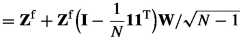(10.10)
\begin{aligned} &= {\mathbf {Z}}^{\mathrm {f}}+ {\mathbf {Z}}^{\mathrm {f}}{\mathbf {W}}/\sqrt{N-1} \end{aligned}
(10.11)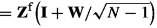(10.12)
\begin{aligned} &= {\mathbf {Z}}^{\mathrm {f}}{\mathbf {T}}, \end{aligned}
(10.13)

with $${\mathbf {W}}$$ defined from Eq. (8.33) as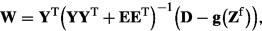(10.14)

and using $$\mathbf {1}^{\mathrm {T}}{\mathbf {W}}=0$$ (Evensen et al., 2019). Thus , using the EnKF update equation it is impossible to obtain an update outside the subspace spanned by the prior ensemble. As in the Topaz system referenced above, the ensemble space may be too restricted to allow for a realistic update incorporating all the measurements’ information. This issue is different from spurious correlations and is directly related to the low rank of the prior ensemble.

### 3.3 Ensemble Representation of the Measurement Information

Another issue with the EnKF update equation is that it effectively projects the measurements onto the ensemble subspace. Let’s use a measurement error covariance matrix $${\mathbf {C}}_{dd}$$ of full-rank and use the Woodbury corollary from Eq. (6.10) to obtain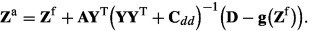(10.15)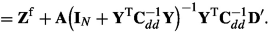(10.16)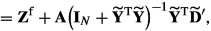(10.17)

where we have defined $$\widetilde{{\mathbf {Y}}} = {\mathbf {C}}_{dd}^{-\frac{1}{2}} {\mathbf {Y}}$$ and $$\widetilde{{\mathbf {D}}}' = {\mathbf {C}}_{dd}^{-\frac{1}{2}} {\mathbf {D}}'$$ which is a normalization or scaling of $${\mathbf {Y}}$$ and $${\mathbf {D}}'$$ by the inverse square root of the measurement error covariance matrix. The important conclusion is that the EnKF update effectively projects the scaled innovations onto the ensemble subspace through the multiplication $$\widetilde{{\mathbf {Y}}}^{\mathrm {T}}\widetilde{{\mathbf {D}}}'$$. Thus, the EnKF update removes all the information in the measurements that the ensemble of predicted measurements cannot represent. This issue is also directly related to the low rank of the prior ensemble.

## 4 Localization in Ensemble Kalman Filters

There are different ways to reduce the impact of sampling errors and the low rank of the prior covariance matrix in ensemble methods. A common approach is to damp the long-range spurious correlations, and  covariance localization (Hamill et al., 2001; Houtekamer & Mitchell, 2001) is one such method. Another alternative is to use the local analysis first used by Haugen and Evensen (2002) and later explained in more detail by Evensen (2003), where one updates variables on subsets of gridpoints using only the nearby observations that we know should impact these variables. In both methodologies, we restrict the influence radius of observations, effectively decoupling regions of the state space far apart. The local analysis allows for different linear combinations of prior ensemble members to be used in the distinct parts of the state space, effectively increasing the prior ensemble-covariance matrix’s rank by orders of magnitude. In a review paper , Sakov and Bertino (2011) discussed the formal similarities between covariance localization and local analysis and concluded that in practice, the two approaches should yield somewhat similar results, and one should base the choice of localization method on criteria such as computational efficiency. We refer to Sakov and Bertino (2011) for an overview of early papers discussing various localization methods, while the more recent review of Chen and Oliver (2017) analyzes different localization scheme when used with an iterative ensemble smoother.

### 4.1 Covariance Localization

In covariance localization, (Anderson, 2003; Bishopetal., 2001; Hamilletal., 2001; Houtekamer & Mitchell, 2001; Whitaker & Hamill, 2002) we use a  damping operator that eliminates long-range spurious correlations in the state covariance matrix $$\overline{{\mathbf {C}}}_{zz}$$. Typically, we would damp each covariance function by multiplying it with a damping function that equals one at the diagonal element and zero at elements corresponding to variables far from the diagonal element. It is common to write the update equations with covariance localization as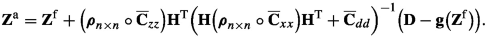(10.18)

Here we have introduced the Schur (or Hadamard) product denoted by $$\circ$$ of elementwise multiplication of two matrices. The matrix of damping functions, $$\boldsymbol{\rho }_{n\times n}$$, acts on the covariance functions in $$\overline{{\mathbf {C}}}_{zz}$$, and uses a scaling equal to one near a measurement and then gradually reduces to zero further away from the measurement location. A commonly used damping function is the one by Gaspari and Cohn (1999) but see also  Furrer and Bengtsson (2007) for an empirical formula that also accounts for the ensemble size. Covariance localization requires us to compute the full state error covariance matrix, which is an overwhelming task for large systems. Since the damping matrix is typically full rank, the Schur product will also be of full rank.

Chen and Oliver (2017) pointed out that with $${\mathbf {H}}$$ being a local and linear measurement operator (rows of $${\mathbf {H}}$$ contains zeros and one element equal to one that corresponds to the measurement), the following applies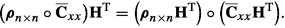(10.19)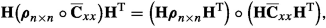(10.20)

Thus, it is possible to replace the Schur product with the $$n\times n$$-dimensional state covariance matrix, by a Schur product with a $$n\times m$$ matrix. Even more importantly, we do not need to form the full state covariance matrix as it suffices to form the covariance matrix between the state variables and the predicted measurements. This observation leads to methods for localization in the observation space.

### 4.2 Localization in Observation Space

For computational efficiency, Houtekamer and Mitchell (2001) proposed to approximate the localization in Eq. (10.18) by writing Eq. (10.1) as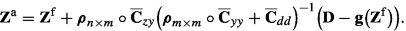(10.21)

We have defined $$\overline{{\mathbf {C}}}_{zy} = \overline{{\mathbf {C}}}_{zz} {\mathbf {H}}^{\mathrm {T}}$$ and $$\overline{{\mathbf {C}}}_{yy} = {\mathbf {H}}\overline{{\mathbf {C}}}_{zz} {\mathbf {H}}^{\mathrm {T}}$$, with $${\mathbf {H}}$$ being a linear measurement operator. With nonlinear measurement operators we can represent the ensemble covariances by their ensemble approximations

\begin{aligned} \overline{{\mathbf {C}}}_{zy}&= {\mathbf {A}}{\mathbf {Y}}^{\mathrm {T}}, \end{aligned}
(10.22)
\begin{aligned} \overline{{\mathbf {C}}}_{yy}&= {\mathbf {Y}}{\mathbf {Y}}^{\mathrm {T}}. \end{aligned}
(10.23)

using the definition in Eqs. (8.3) and (8.8). As pointed out by Chen and Oliver (2017), the relations in Eqs. (10.19) and (10.20) are not valid for general observation operators. Thus, we need to define $$\boldsymbol{\rho }_{n\times m}$$ and $$\boldsymbol{\rho }_{m\times m}$$ according to the problem at hand.

Chen and Oliver (2017) also discussed Kalman-gain localization where one localizes the Kalman-Gain matrix directly as

\begin{aligned} {\mathbf {Z}}^{\mathrm {a}}= {\mathbf {Z}}^{\mathrm {f}}+ \boldsymbol{\rho }_{n\times m} \circ {\mathbf {K}}\Bigl ({\mathbf {D}}- {\mathbf {g}}\bigl ({\mathbf {Z}}^{\mathrm {f}}\bigr ) \Bigr ). \end{aligned}
(10.24)

Similarly to Eq. (10.25) we must compute the full $$\overline{{\mathbf {C}}}_{zy} \in \Re ^{n\times m}$$, but we ignore the localization of $$\overline{{\mathbf {C}}}_{yy}$$. Kalman-gain localizaion is popular in the petrolium community and does indeed reduce the impact of spurious correlations. However, it does not remove the spurious correlations in $${\mathbf {C}}_{yy}$$ that induce unphysical dependencies between remote meaurements and thereby reduces their impact on the update.

### 4.3 Localization in Ensemble Space

When using Eqs. (10.22) and (10.23) we can write Eq. (10.21) as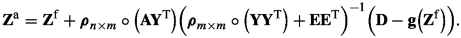(10.25)

Thus, we can extend this discussion to search for a localization approach in the ensemble subspace where the Schur product acts directly on the ensemble anomalies. As for the Kalman-gain localization, we neglect the localization of the covariance of the predicted measurement anomalies. Thus, we write Eq. (10.25) as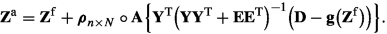(10.26)

However, in this formulation, we need to redefine our interpretation of the data and represent them in the ensemble subspace. Following the derivation in Eqs. (10.15)–(10.17) we have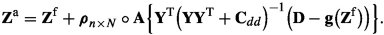(10.27)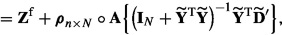(10.28)

with $$\widetilde{{\mathbf {Y}}} = {\mathbf {C}}_{dd}^{-\frac{1}{2}} {\mathbf {Y}}$$ and $$\widetilde{{\mathbf {D}}}' = {\mathbf {C}}_{dd}^{-\frac{1}{2}} {\mathbf {D}}'$$ as before. Thus, by projecting the measurement innovations onto the ensemble subspace through the product $$\widetilde{{\mathbf {Y}}}^{\mathrm {T}}\widetilde{{\mathbf {D}}}'$$, we approximately represent the measurement’s information by N projected measurements. Note that it is not possible to use a physical distance in the localization scheme for the N projected measurements. However, the adaptive localization schemes discussed below might be used in this case. The main problem with this approach is the following: one reason for applying localization is that the measurements contain more information than the ensemble subspace can accommodate. Thus, localization allows us to compute a more prosperous update that is not confined to the ensemble subspace. But, from Eq. (10.28), we project the original measurements onto the ensemble subspace, and we thereby lose all measured information that the ensemble subspace cannot represent. So, in this case, why would we localize at all? But, see also the ideas suggested by Buehner (2005).

### 4.4 Local Analysis

Brusdal et al. (2003)  and Haugen and Evensen (2002) used  a distance-based local-analysis scheme in an ocean circulation model, where for each vertical column of gridpoints, they updated the state variables using only nearby-located measurements, we quote from Haugen and Evensen (2002) “Note that only data located at gridpoints within a certain influence radius (here chosen to 40 km) are used in the update of the state variables in each gridpoint. This is a common procedure normally denoted as a local analysis.” Evensen (2003) gave a more detailed explanation of the local analysis , and later, Ott et al. (2004) introduced the  popular local ensemble transform Kalman filter (LETKF) using the same local-analysis concept.

In local analysis, we first need to select subsets of variables to update independently. In principle, we could update the elements in the state vector one by one, but that will generally become too computationally expensive. So instead, a sensible approach is to select all variables associated with a vertical column of gridpoints. Alternatively, if vertical localization is essential, we can split the model grid into subgroups of grid points with a limited horizontal and vertical extent. After that, we must select which measurements to include in the analysis update for each subgroup. We can often use a distance-based approach to retain all the measurements located within a specific range from the subgroup to be updated. However, in some applications, we have so-called non-local measurements where the measured information results from non-local physical processes that can extend over large parts of the model domain. One such example includes pressure transients between wells in reservoir models. Adaptive localization may be a better alternative in these cases. We then select measurements based on their correlation with a group of variables being significant  (Neto et al., 2021).

When using local analysis, we can write the update equation Eq. (10.5) in the three following forms

\begin{aligned} {\mathbf {Z}}_{l}^{\mathrm {a}}&={\mathbf {Z}}_{l}^{\mathrm {f}}+ {\mathbf {A}}_{l} {\mathbf {W}}_{l} \end{aligned}
(10.29)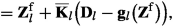(10.30)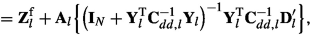(10.31)

where l runs over the different subgroups of local model variables. Here $${\mathbf {W}}_l$$ and $$\overline{{\mathbf {K}}}_l$$ are local variants of Eqs. (10.14) and (10.7) evaluated using the selected observations for each l. As each local update uses an individual Kalman gain or weight matrix, the updated ensemble will no longer be confined to the prior ensemble space. Note that the local analyses are straightforward to parallelize as the computations for different values of l are independent.

There is a computational advantage of using local analysis rather than covariance (or Kalman gain) localization when working with ensemble methods. The reason is that the Kalman-gain matrix is of low rank unless the number of measurements is less than the number of ensemble realizations. Thus, forming the Kalman gain matrix and performing a Schur product is significantly more computationally demanding than computing the local analysis. This statement is true even though it is possible to calculate the Kalman gain matrix and the corresponding update row by row in parallel (Chen & Oliver, 2017). However, in the local analysis it is not uncommon to have $$n \sim m \sim N$$, and when the ratio m/N is sufficiently small, the Kalman gain update in Eq. (10.30) becomes more computational efficient than the update in Eq. (10.29).

A variant of the local-analysis scheme combines the formulas in Eq. (10.30) with the tapering used for covariance or Kalman gain localization  (Chen & Oliver, 2017). This approach was also applied by  Neto et al. (2021). Alternatively, the local analysis with observation taper (Greybush et al., 2011; Hunt et al., 2007)  uses the form Eq. (10.31) when computing the local updates and then tapers the (in their case diagonal) inverse of the error covariance matrix $${\mathbf {C}}^{-1}_{dd}$$ for each local update. When using the local analysis in the form Eq. (10.29), we can inflate the variance of the remotest located measurements by scaling selected rows in $${\mathbf {E}}$$ to obtain the same effect. The tapering of the local updates reduces the impact of the local measurements located furthest from the gridpoints being updated and reduces the discontinuities in the updated solution. Note that covariance tapering of the local analysis updates is affordable since both the local state dimension and the number of measurements are very low compared to the global analysis update.

In cases with non-local measurements, where it is impossible to use distance-based tapering, it might be possible to use an adaptive localization method. In adaptive localization, we use the ensemble correlations between a predicted measurement and the state variables at a particular gridpoint to determine if we should update these variables using this measurement. The most straightforward approach is to truncate all observations that have correlations below a certain level. This approach eliminates the impact of spurious correlations but also removes weak but physical correlations. To improve on this approach, Anderson (2007b) proposed using many small ensembles to check if correlations are significant, while Bishop and Hodyss (2007) uses the correlation function to derive a tapering function.  Fertig et al. (2007) used the ensemble correlation function to decide whether to update a variable or not. Evensen (2009b, Chap. 15) discussed adaptive localization based on the truncation of small covariances in an example with an advection equation. He found that the approach worked well but led to small discontinuities in the updates, which will likely cause problems in many nonlinear models. Luo et al. (2019) and Luo and Bhakta (2020) have continued this work and developed tuned schemes where they combine truncation-based adaptive localization with tapering of each local update . Neto et al. (2021) and Soares et al. (2021) used this approach successfully in petroleum applications. It is essential to focus the localization issue of non-local observations, not on the prior covariance itself, but the state-observation correlations. As Van Leeuwen (2019) shows, non-local measurements can influence distant state variables, not physically connected in the prior covariance, but they become connected via the observation operator.

## 6 Localization in Time

Localization also plays an important role when using iterative ensemble smoothers like EnRML and ESMDA. Particularly when we use iterative ensemble smoothers in sequential data assimilation, the accumulation of errors resulting from spurious correlations will impact the results significantly. It becomes more tricky to define which observations should impact which state variables when we localize in time. For instance, we know that the information propagates on the model characteristics for hyperbolic models, such as the linear advection equation. In this case, when updating the solution at a gridpoint, we should include all measurements located close to the characteristic line intersecting this grid point. For more complex nonlinear models, the situation becomes even more complicated.

On the other hand, many realistic models have chaotic behavior, limiting the time interval over which measurements will impact the update. There are several proposed solutions. The simplest is to use a larger influence radius with time localization to include all relevant observations as, e.g., used in  Brusdal et al. (2003). Bocquet  (2015) discusses several time localization schemes where the localization domain effectively propagates with the dynamical flow. Amezcua et al. (2017)  show how a weak-constraint ensemble smoother strongly reduces the severity of the issue because the model errors can absorb observation influences local in time and transfer them to the state variables that propagate through time. But maybe adaptive localization will be even more helpful in the case of localization in time.

## 7 Inflation

Anderson and Anderson (1999) suggested using an approach named inflation to counteract the excessive variance reduction caused by spurious correlations in the update. Inflation is generally needed to avoid filter divergence in operational ensemble data-assimilation systems with small ensemble sizes. We can implement inflation as a scaling of the ensemble anomalies

\begin{aligned} {\mathbf {A}}\leftarrow \rho {\mathbf {A}}, \end{aligned}
(10.32)

where $$\rho$$ is a factor slightly larger than one. Today, most operational ensemble data-assimilation systems apply some calibrated inflation to counteract different error sources. We can inflate before or after the analysis update. If applied to the forecast ensemble, inflation is a way to account for model errors and compensate for the low-rank approximation, increasing the predicted ensemble variance. If we inflate the analysis ensemble, inflation accounts for errors introduced by the analysis scheme, e.g., spurious correlations, the approximate representation of the measurement error covariance matrix, and possibly adverse localization effects. The standard procedure is to inflate the analysis update and calibrate the inflation factor to obtain a data-assimilation system in good agreement with observations. As such, inflation is an approach that tries to correct “everything” wrong in the system.

Some papers attempt to estimate an optimal inflation parameter adaptively. Anderson (2009) proposed a method for adaptively estimating a spatially and temporally varying inflation parameter using a Bayesian algorithm. The algorithm is recursive and updates the inflation parameter with time. Wang and Bishop (2003) uses the sequence of innovation statistics to compute the covariance inflation, while Anderson (2007a) estimates the inflation parameter as part of the state vector. Sacher and Bartello (2008) discuss the sampling errors in EnKF and proposes an analytical expression for the optimal covariance inflation method, which depends on the Kalman gain, the analyzed variance, and the number of realizations. But see also the adaptive inflation estimation by Evensen (2009b) targeting the impact of spurious correlations.

## 8 Localization in Particle Filters

In particle filters, we introduce localization for a different reason than in ensemble Kalman filter methods. As we have seen in Chap. 9, particle filters do not rely on accurate estimation of covariance matrices, which is essential for the success of variational methods and (iterative) ensemble Kalman filters. The problem here is that the weights are degenerate when the number of independent observations  is large, see, e.g., Ades and Van Leeuwen (2015a), and Snyder et al. (2008, 2015). And “large” is minor for geophysical applications, where more than ten independent observations typically force us to use tens of thousands of particles. Hence, we use localization in particle filters to reduce the number of measurements in the likelihood of each gridpoint.

The idea of using localization in particle filters was first introduced in 2003 in three  papers (Bengtsson et al., 2003; Van Leeuwen 2003a, b). Localization in particle filters faces two main problems. One issue with localization is that different sets of particles will survive after resampling different gridpoints. It is hard to connect these different particle sets from different gridpoints to form smooth global particles that the model equations can propagate. Practical solutions all diminish the influence of the observations, e.g., by setting a minimum weight for each particle, which restricts the size of the update of the prior particles (Poterjoy, 2016; Poterjoy & Anderson, 2016), or reducing the observation space to that of the ensemble space of the prior particles before calculating weights  Potthast et al. (2019), or combinations of these. Typically, further smoothing is needed, such as relaxation to prior particles.

Another localization issue is that in some geophysical systems, such as the atmosphere, the number of measurements inside the localization radius will still be too large, and the filter becomes degenerate. The point here is that the localization radius should be connected to physical length scales to consider all relevant observations for a gridpoint. However, for a global atmospheric model, the order of the localization radius is 1000 km, the typical size of a low-pressure area, which often contains millions of observations. The only serious solution to this problem is to project the observations onto the ensemble space, but that still does ignore large parts of the observation information.

Of course, this problem does not exist when one does pure parameter estimation, and localization can be a beneficial technique. The first to explore this is  Vossepoel and Van Leeuwen, (2007), who used 128 particles to successfully update the order of 10,000 turbulence parameters in a global ocean model.

## 9 Summary

Localization allows us to compute an updated ensemble with realizations outside the space spanned by the initial ensemble. We have discussed several localization methods in this chapter, and the most efficient methods depend on the problem at hand. Localization and inflation are essential tools in high-dimensional data assimilation problems. They are, in practice, used for many other issues than the low-rank prior covariance and spurious correlations between gridpoints far apart. They are also used as tuning parameters to compensate for many problems, such as unknown model errors, approximations in data-assimilation schemes, forward model deficiencies, less well-known observation operators, etc.

Both localization and inflation are, in essence, ad-hoc procedures invented to make the system work. As such, they can introduce so-called unbalanced system states. The classic example is a linear model, where all realizations are solutions, and consequently, linear combinations of the realizations will also be solutions. When applying localization, we break this property and introduce realizations that may not be physically realizable or strong adjustment dynamics to the unbalanced part of the system’s state space.

Finally, localization and inflation are two approximate methods to correct related errors in the data assimilation system. Therefore, we must calibrate them to work complementary together.